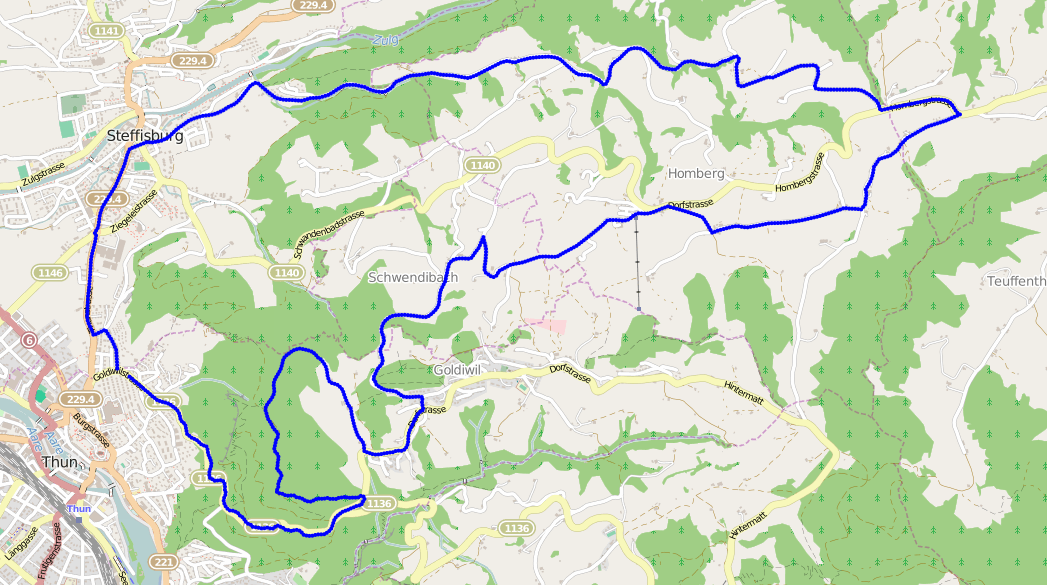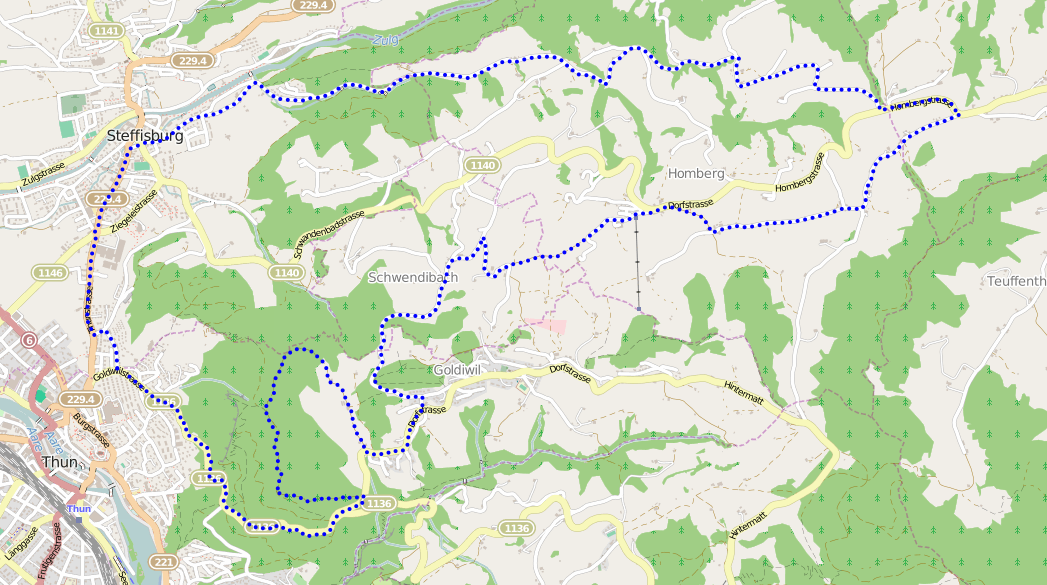# How to distribute GPS points evenly

In How to compare GPS tracks I showed how the Needleman-Wunsch algorithm, originally designed for aligning DNA and protein sequences, can be used to compare GPS tracks.The method I introduced relies on pre-processing the GPS file so that all points are evenly distributed to reduce noise in the data set, but I didn’t cover how that’s done.

# Trace the path

Starting from the first point, the path is followed and the great-circle distance between each point is accumulated until it exceeds the desired distribution distance.

When the distance has been exceeded, a new point is interpolated between the current point and the previous point.

gpxpy has a function for moving points when given an angle and a distance in meters. The distance is the accumulated distance minus the required distance. gpxpy doesn’t have a function for calculating the angle, otherwise known as initial bearing, between two points. Using the information here, I implemented the following function:

``````1def bearing(point1, point2):
4    dlon = math.radians(point2.longitude - point1.longitude)
5
6    y = math.sin(dlon) * math.cos(lat2r)
7    x = math.cos(lat1r) * math.sin(lat2r) - math.sin(lat1r) \
8                        * math.cos(lat2r) * math.cos(dlon)
9    return math.degrees(math.atan2(y, x))
``````

# Interpolate points

Points are interpolated between the last correct point and current point until the distance no longer exceeds the distribution distance, then the entire process repeats until the end of the track is reached. The final point is always retained.

`````` 1def interpolate_distance(points, distance):
2    d = 0
3    i = 0
4    even_points = []
5    while i < len(points):
6        if i == 0:
7            even_points.append(points)
8            i += 1
9            continue
10
11        if d == 0:
12            p1 = even_points[-1]
13        else:
14            p1 = points[i-1]
15        p2 = points[i]
16
17        d += gpxpy.geo.distance(p1.latitude, p1.longitude, p1.elevation,
18                                p2.latitude, p2.longitude, p2.elevation)
19
20        if d >= distance:
21            brng = bearing(p1, p2)
22            ld = gpxpy.geo.LocationDelta(distance=-(d-distance), angle=brng)
23            p2_copy = copy.deepcopy(p2)
24            p2_copy.move(ld)
25            even_points.append(p2_copy)
26
27            d = 0
28        else:
29            i += 1
30    else:
31        even_points.append(points[-1])
32
33    return even_points
``````

# Results

The result from using this algorithm on the same data produces a much more spectacular result:And just in case you don’t believe me, here’s the same data with points distributed every 50 meters:The code can be found in jonblack/cmpgpx repository on GitHub.